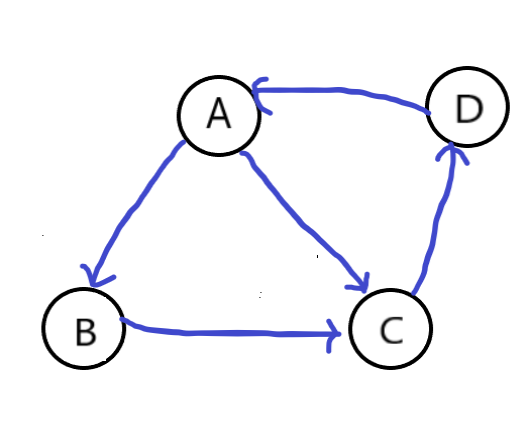# 网页排名算法PageRank

## 1.简单PR模型$PR(u) = \sum_{v\in IN_{u}} \frac{PR(v)}{N(v)}$

$M_{A}=\begin{bmatrix}0\\1/2\\1/2\\0\\ \end{bmatrix}$

$M=\begin{bmatrix}0 & 0 & 0 & 1\\ 1/2 & 0 & 0 & 0\\ 1/2 & 1 & 0 & 0\\ 0 & 0 & 1 & 0\\ \end{bmatrix}$

$W_{0} =\begin{bmatrix}1/4\\1/4\\1/4\\1/4\\ \end{bmatrix}$

$W_{1} =\begin{bmatrix}0 & 0 & 0 & 1\\ 1/2 & 0 & 0 & 0\\ 1/2 & 1 & 0 & 0\\ 0 & 0 & 1 & 0\\ \end{bmatrix}\begin{bmatrix}1/4\\1/4\\1/4\\1/4\\ \end{bmatrix}=\begin{bmatrix}0.25\\0.125\\0.375\\0.25 \end{bmatrix}$

$W_{n} =\begin{bmatrix}0 & 0 & 0 & 1\\ 1/2 & 0 & 0 & 0\\ 1/2 & 1 & 0 & 0\\ 0 & 0 & 1 & 0\\ \end{bmatrix}^n \begin{bmatrix}1/4\\1/4\\1/4\\1/4\\ \end{bmatrix} =\begin{bmatrix}0.28613281\\0.14367676\\0.28588867\\0.28430176 \end{bmatrix}$

import numpy as np

#简易版
def pageRank1(G,delta):
#G为一个方阵，描述了结点间的游走概率
#delta为可接受误差，只要迭代过程中，前后两次算出来的权重列向量的差方小于delta，就认为收敛了
n = G.shape  #n为矩阵行数（结点个数）
w = np.ones(n)*(1/n)   #初始化每个页面的权重，w是一个长为n的列向量，每个元素代表该网页的权重，初始值为1/n
maxIter = 1000   #最大迭代次数为1000次
for i in range(0,maxIter):
tw = w       #暂存上次记录
w = np.dot(G,w)     #计算新的概率
err = np.power((tw-w),2)  #做差取平方
if(sum(err)<delta): #若收敛了，就停止迭代
break
return w          #返回计算的结果



## 2.修正的PR模型

$PR(u) = (1-d) + d\sum_{v\in IN_{u}} \frac{PR(v)}{N(v)}$

#修正版
def pageRank2(G,D,E=0.00001):
#D是阻尼系数
#E是可接受误差
n = G.shape  #n为矩阵行数（结点个数）
w = np.ones(n)*(1/n)
maxIter = 1000   #最大迭代次数为1000次
for i in range(0,maxIter):
tw = w       #暂存上次记录
w = (1-D)/ n + D * np.dot(G, w)     #计算新的概率
err = np.power((tw - w), 2)  #做差取平方
if(sum(err)<E):
break
return w



## 参考资料：

【1】机器学习经典算法之PageRank https://www.cnblogs.com/jpcflyer/p/11180263.html